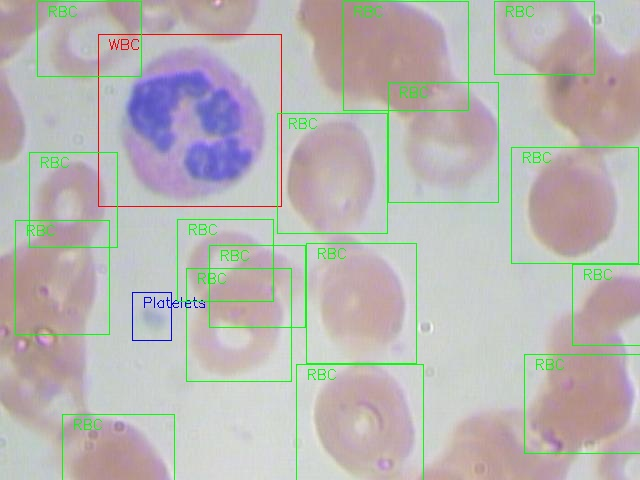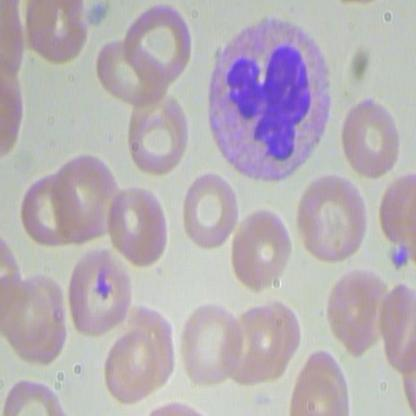# Train an object detection model with Vertex AI AutoML and Kili for faster annotation

## What is Google Vertex AI?

Vertex AI is a comprehensive machine learning platform designed for the training, deployment, and customization of ML models and AI applications, including large language models (LLMs). It seamlessly integrates with Kili to create a sophisticated model-in-the-loop data annotation workflow.

For more information on Google Vertex AI AutoML, you can visit Introduction to Vertex AI and the AutoML beginner's guide.

## Tutorial Objectives

In this tutorial, we will demonstrate how to train an object detection model with annotated data on Google Vertex AI AutoML and utilize it to make predictions on unlabeled data to speed up the annotation process. The tutorial will cover the following steps: 1. Fetching and preparing data from Kili 2. Training a model with Vertex AI 3. Running predictions on unlabeled images from your Kili dataset 4. Uploading predictions to our Kili project for faster annotation

All steps can be performed through the Google Cloud Console UI, but this tutorial focuses on performing actions exclusively through the AI Platform Python SDK and Kili Python SDK to provide a deeper integration.

For this tutorial, we will use the BCCD dataset (Blood Cell Count and Detection), which is accessible on GitHub and also hosted on Hugging Face or Roboflow. It consists of 3 highly unbalanced classes: platelets, RBCs (red blood cells), and WBCs (white blood cells).## Installation Requirements

!pip install -U google-cloud-aiplatform
!pip install kili

import json
import mimetypes
import random
from pathlib import Path
from typing import List, Union

import requests
from PIL import Image
from tqdm import tqdm

from kili.client import Kili


We first initialize the Kili client.

The API Key can be found in the settings of your project, in the "interface" section.. The object detection job name of your project can be found in the interface of your project.

kili_api_key = "[KILI API KEY]"
project_id = "[KILI PROJECT ID]"
OBJECT_DETECTION_JOB_NAME = "[OBJECT DETECTION JOB NAME]"  # e.g: OBJECT_DETECTION_JOB

kili = Kili(api_key=kili_api_key)

# Authenticate with Google Cloud
auth.authenticate_user()

# Initialize the GCS client
storage_client = storage.Client()

project_name = "[GCP PROJECT NAME]"
location = "[GCP PROJECT LOCATION]"
bucket_name = "[GCS BUCKET NAME]"
dataset_name = "blood_cell"  # name of the dataset you will create in Vertex AI
bucket_dataset_dir = (
f"experiments/{dataset_name}"  # name of the folder in yout bucket where files will be stored.
)

aiplatform.init(project=project_name, location=location)


## Prepare the Data

To train our Vertex AI AutoML model, we need to prepare the data within our Kili project. This section follows the guidelines provided in the Vertex AI data preparation documentation

This section covers the following steps: - Downloading annotated images from Kili to your local machine along with their labels. - Uploading the images to Google Cloud Storage. - Splitting the annotated data into train/validation/test sets. - Converting the images and labels into the required format for Vertex AI datasets. - Uploading the converted input data to Google Cloud Storage.

In this particular example, we have annotated 150 assets on the Kili app.

We first call the Kili Python SDK assets function in order to retrieve assets.

The download_media argument allows to download the media (images here) in the folder given in the local_media_dir argument. When doing so, the content field will automatically be replaced by the local path of the downloaded asset.

For each asset, we query its id, externalId, and the jsonResponse fields of its latest label (the last one submitted on Kili). For more information on the assets function or on other fields that you can query, you can have a look at the function documentation.

assets = kili.assets(
project_id=project_id,
local_media_dir="./images",
status_in=["LABELED"],
fields=["latestLabel.jsonResponse", "content", "id", "externalId"],
disable_tqdm=False,
)

# Plot an example image
Image.open(assets["content"])When importing data to a Vertex AI Datset, the images must already be stored on a Google Cloud Storage:

def upload_assets_to_bucket(assets: List[dict], bucket_name: str, bucket_dataset_dir: str):
bucket = storage_client.get_bucket(bucket_name)
image_bucket_path = f"{bucket_dataset_dir}/images/{Path(asset['content']).name}"
image_local_path = asset["content"]
blob = bucket.blob(image_bucket_path)

upload_assets_to_bucket(assets, bucket_name, bucket_dataset_dir)


### Splitting the Dataset into Train/Validation/Test Sets

We will divide our annotated images using the following proportions:

• Training set: 70%
• Validation set: 20%
• Test set: 10%
def split_assets_in_train_val_test(assets: list[dict]):
# shuffle the assets before splitting
random.shuffle(assets)

# Calculate the lengths of each split
total_len = len(assets)
train_len = int(0.7 * total_len)
val_len = int(0.2 * total_len)

# Split the list into train, validation, and test
train_assets = assets[:train_len]
val_assets = assets[train_len : train_len + val_len]
test_assets = assets[train_len + val_len :]

return train_assets, val_assets, test_assets

train_assets, val_assets, test_assets = split_assets_in_train_val_test(assets)
print(len(train_assets), len(val_assets), len(test_assets))

105 30 15


### Converting images and labels into Vertex AI required format

Data that we upload to the Vertex AI dataset must follow a predefined schema. The schema is provided in a YAML file format that is based on the OpenAPI format.

In the following function, we generate JSON data that adheres to the required schema for an asset. This includes the location of the asset on Google Cloud Storage, the bounding boxes with their coordinates and categories, as well as the asset split.

def get_asset_io_input(asset: list[dict], split: str, bucket_name: str, image_bucket_path: str):
image_gcs_uri = f"gs://{bucket_name}/{image_bucket_path}"
labels = []
for annotation in asset["latestLabel"]["jsonResponse"][OBJECT_DETECTION_JOB_NAME][
"annotations"
]:
normalizedVertices = annotation["boundingPoly"]["normalizedVertices"]
label = {
"displayName": annotation["categories"]["name"],
"xMin": min(map(lambda vertice: vertice["x"], normalizedVertices)),
"yMin": min(map(lambda vertice: vertice["y"], normalizedVertices)),
"xMax": max(map(lambda vertice: vertice["x"], normalizedVertices)),
"yMax": max(map(lambda vertice: vertice["y"], normalizedVertices)),
}
labels.append(label)

return {
"imageGcsUri": image_gcs_uri,
"boundingBoxAnnotations": labels,
}


We will generate one jsonl file storing all image json input. Each line of the jsonl file will corresponds to one data to import in the previously defined format

This jsonl file then needs to be imported to the Google Cloud Storage bucket and will be the input given at the dataset creation.

def generate_and_upload_inputs_to_bucket(
assets: List[dict], bucket_name: str, bucket_dataset_dir: str
):
output_jsonl_file = "inputs.jsonl"
bucket = storage_client.get_bucket(bucket_name)
with open(output_jsonl_file, "w") as output_file:
for split_name, split_assets in [
("training", train_assets),
("validation", val_assets),
("test", test_assets),
]:
for asset in split_assets:
# get the input object to be sent when importing the dataset
image_bucket_path = f"{bucket_dataset_dir}/images/{Path(asset['content']).name}"
input = get_asset_io_input(asset, split_name, bucket_name, image_bucket_path)

# add the example input to the jsonl file
json_line = json.dumps(input)
output_file.write(json_line + "\n")

# upload the inputs file to the bucket
blob = bucket.blob(f"{bucket_dataset_dir}/inputs.jsonl")

generate_and_upload_inputs_to_bucket(assets, bucket_name, bucket_dataset_dir)


## Create a dataset in Vertex

To train an autoML model, we need a Vertex AI dataset.

When creating this dataset, we also provide the jsonl file generated in the last section to import images with their labels into the dataset.

For more information on dataset creation, you can have a look at Vertex AI documentation

def create_and_import_dataset_image_sample(
project: str,
location: str,
display_name: str,
src_uris: Union[str, List[str]],
import_schema_uri: str,
sync: bool = True,
):
aiplatform.init(project=project, location=location)

ds = aiplatform.ImageDataset.create(
display_name=display_name,
gcs_source=src_uris,
import_schema_uri=import_schema_uri,
sync=sync,
)

ds.wait()

print(ds.display_name)
print(ds.resource_name)
return ds

inputs_uri = f"gs://{bucket_name}/{bucket_dataset_dir}/inputs.jsonl"
import_schema_uri = (
)
ds = create_and_import_dataset_image_sample(
project_name, location, dataset_name, inputs_uri, import_schema_uri
)

INFO:google.cloud.aiplatform.datasets.dataset:Creating ImageDataset
INFO:google.cloud.aiplatform.datasets.dataset:To use this ImageDataset in another session:

dataset_id = ds.name.split("/")[-1]

2314474175491735552